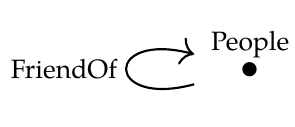>Puzzle 122. Let C be the free category on this graph:

>>Let's use this as a database schema. Draw an example of a database built using this schema. Mathematically it's a functor F:C→Set. But what does this mean in concrete terms? You can draw it as a table, like you would see in a spreadsheet.

Giving an instance to the above free-category \$$C\$$, just produces something that looks like a 2 column table,

\$\begin{array}{c|c} \text{People} & \mathrm{FriendOf} \\\\ \hline Alice & Bob \\\\ Bob & Alice \\\\ \vdots & \vdots \\\\ Stan & Tyler \\\\ Tyler & Stan. \\\\ \vdots & \vdots \end{array} \$